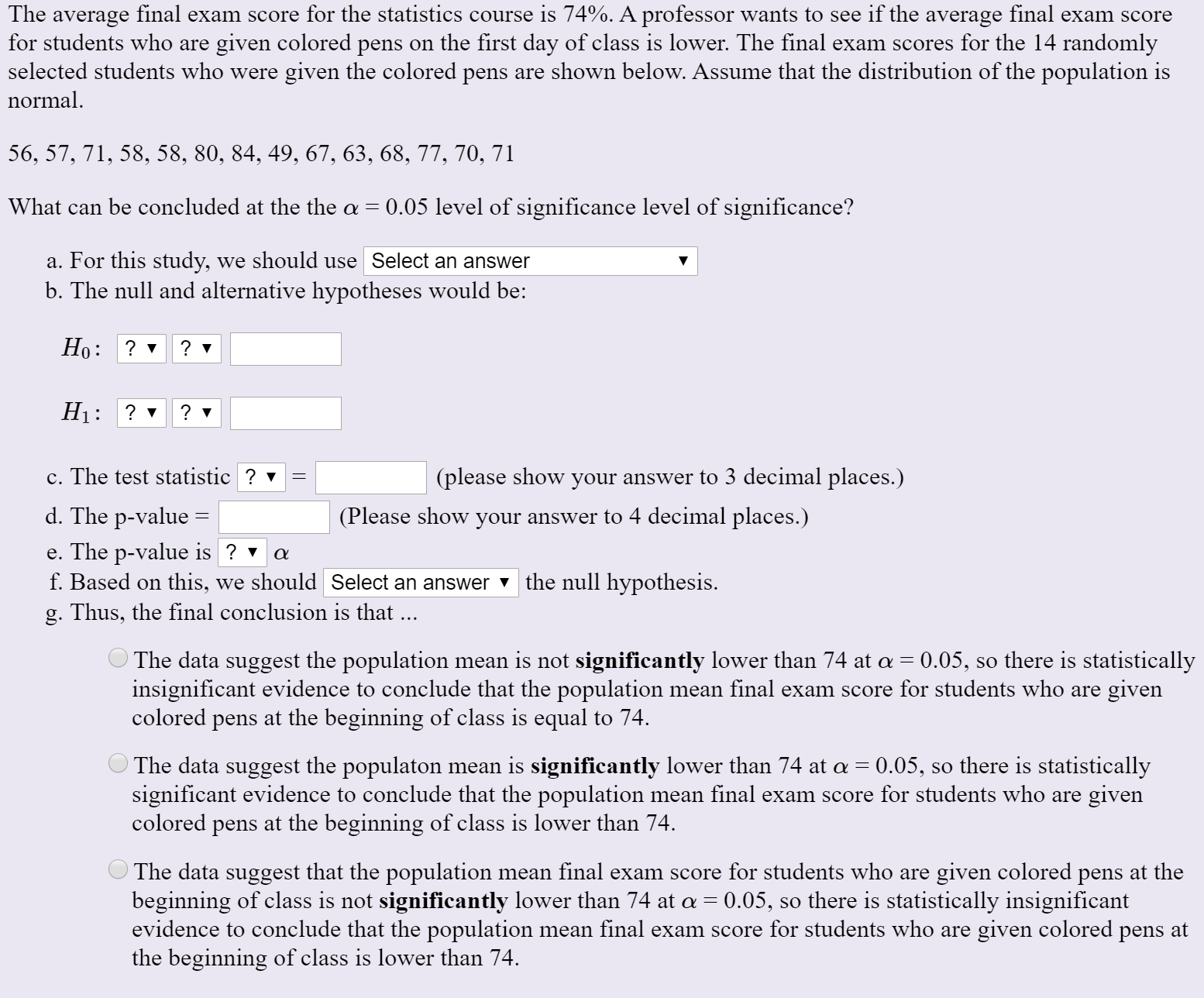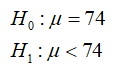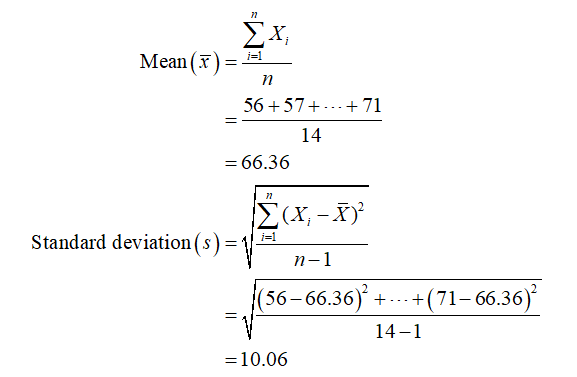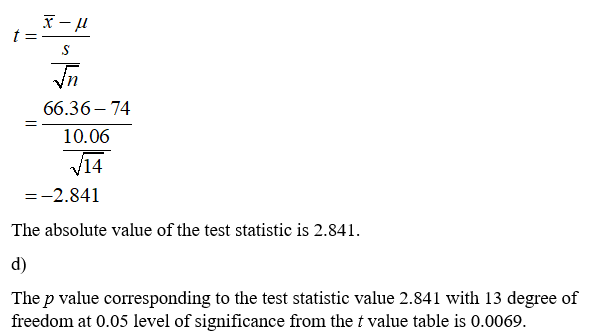# The average final exam score for the statistics course is 74%. A professor wants to see if the average final exam scorefor students who are given colored pens on the first day of class is lower. The final exam scores for the 14 randomlyselected students who were given the colored pens are shown below. Assume that the distribution of the population isnormal.56, 57, 71, 58, 58, 80, 84, 49, 67, 63, 68, 77, 70, 71What can be concluded at the the a = 0.05 level of significance level of significance?a. For this study, we should use Select an answerb. The null and alternative hypotheses would be:Но: ?H1:? ▼? vc. The test statistic ? v(please show your answer to 3 decimal places.)d. The p-value =(Please show your answer to 4 decimal places.)e. The p-value is ? vf. Based on this, we should Select an answer the null hypothesis.g. Thus, the final conclusion is that ...The data suggest the population mean is not significantly lower than 74 at a = 0.05, so there is statisticallyinsignificant evidence to conclude that the population mean final exam score for students who are givencolored pens at the beginning of class is equal to 74.The data suggest the populaton mean is significantly lower than 74 at a = 0.05, so there is statisticallysignificant evidence to conclude that the population mean final exam score for students who are givencolored pens at the beginning of class is lower than 74.The data suggest that the population mean final exam score for students who are given colored pens at thebeginning of class is not significantly lower than 74 at a = 0.05, so there is statistically insignificantevidence to conclude that the population mean final exam score for students who are given colored pens atthe beginning of class is lower than 74.

Question
7 viewshelp_outlineImage TranscriptioncloseThe average final exam score for the statistics course is 74%. A professor wants to see if the average final exam score for students who are given colored pens on the first day of class is lower. The final exam scores for the 14 randomly selected students who were given the colored pens are shown below. Assume that the distribution of the population is normal. 56, 57, 71, 58, 58, 80, 84, 49, 67, 63, 68, 77, 70, 71 What can be concluded at the the a = 0.05 level of significance level of significance? a. For this study, we should use Select an answer b. The null and alternative hypotheses would be: Но: ? H1: ? ▼ ? v c. The test statistic ? v (please show your answer to 3 decimal places.) d. The p-value = (Please show your answer to 4 decimal places.) e. The p-value is ? v f. Based on this, we should Select an answer the null hypothesis. g. Thus, the final conclusion is that ... The data suggest the population mean is not significantly lower than 74 at a = 0.05, so there is statistically insignificant evidence to conclude that the population mean final exam score for students who are given colored pens at the beginning of class is equal to 74. The data suggest the populaton mean is significantly lower than 74 at a = 0.05, so there is statistically significant evidence to conclude that the population mean final exam score for students who are given colored pens at the beginning of class is lower than 74. The data suggest that the population mean final exam score for students who are given colored pens at the beginning of class is not significantly lower than 74 at a = 0.05, so there is statistically insignificant evidence to conclude that the population mean final exam score for students who are given colored pens at the beginning of class is lower than 74. fullscreen
check_circle

Step 1

a)

For this study one tailed single sample t- test will be used.

From the provided information,

b)

The null and alternative hypotheses can be constructed as:Sample size (n) = 14

The degree of freedom = 14 – 1 = 13

The mean and standard deviation can be obtained as:Step 2

c)

The test statistic can be obtained as:...

### Want to see the full answer?

See Solution

#### Want to see this answer and more?

Solutions are written by subject experts who are available 24/7. Questions are typically answered within 1 hour.*

See Solution
*Response times may vary by subject and question.
Tagged in